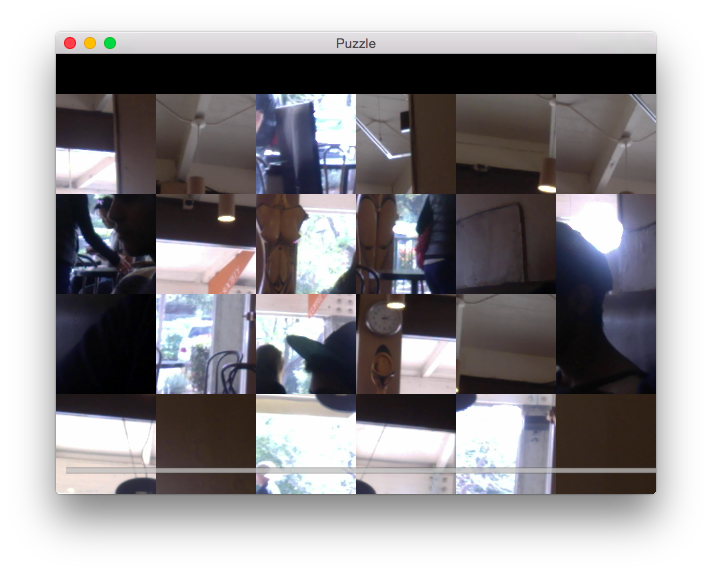# Shuffled Camera Feed Puzzle¶This demonstrates using Scatter widgets with a live camera. You should see a shuffled grid of rectangles that make up the camera feed. You can drag the squares around to see the unscrambled camera feed or double click to scramble the grid again.

## File demo/camera_puzzle.py¶

'''
Shuffled Camera Feed Puzzle
===========================

This demonstrates using Scatter widgets with a live camera.
You should see a shuffled grid of rectangles that make up the
camera feed. You can drag the squares around to see the
unscrambled camera feed or double click to scramble the grid
again.
'''

from kivy.app import App
from kivy.uix.camera import Camera
from kivy.uix.widget import Widget
from kivy.uix.slider import Slider
from kivy.uix.scatter import Scatter
from kivy.animation import Animation
from kivy.graphics import Color, Rectangle
from kivy.properties import NumericProperty
from random import randint, random
from functools import partial

class Puzzle(Camera):

blocksize = NumericProperty(100)

def on_texture_size(self, instance, value):
self.build()

def on_blocksize(self, instance, value):
self.build()

def build(self):
self.clear_widgets()
texture = self.texture
if not texture:
return
bs = self.blocksize
tw, th = self.texture_size
for x in range(int(tw / bs)):
for y in range(int(th / bs)):
bx = x * bs
by = y * bs
subtexture = texture.get_region(bx, by, bs, bs)
# node = PuzzleNode(texture=subtexture,
#                  size=(bs, bs), pos=(bx, by))
node = Scatter(pos=(bx, by), size=(bs, bs))
with node.canvas:
Color(1, 1, 1)
Rectangle(size=node.size, texture=subtexture)

self.shuffle()

def shuffle(self):
texture = self.texture
bs = self.blocksize
tw, th = self.texture_size
count = int(tw / bs) * int(th / bs)
indices = list(range(count))
childindex = 0
while indices:
index = indices.pop(randint(0, len(indices) - 1))
x = bs * (index % int(tw / bs))
y = bs * int(index / int(tw / bs))
child = self.children[childindex]
a = Animation(d=random() / 4.) + Animation(pos=(x, y),
a.start(child)
childindex += 1

def on_touch_down(self, touch):
if touch.is_double_tap:
self.shuffle()
return True
super(Puzzle, self).on_touch_down(touch)

class PuzzleApp(App):
def build(self):
root = Widget()
puzzle = Puzzle(resolution=(640, 480), play=True)
slider = Slider(min=100, max=200, step=10, size=(800, 50))
slider.bind(value=partial(self.on_value, puzzle))